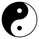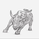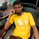17488 obejrzeń
17488
Took the code from LazyBears rsi-fib and made it so you could apply it to a chart. It plots fib levels between the high and low of a timeframe of your choosing. Seems to line up well with custom drawn fib levels.

Not sure if it's any better than just drawing the lines yourself, but whatever.
```study(title="FibFib", shorttitle="AutoFib", overlay=true)
fiblength=input(265)
maxr = highest(close, fiblength)
minr = lowest(close, fiblength)
ranr = maxr - minr

ON=plot( maxr , color=black,  title="1")
SS=plot( maxr - 0.236 * ranr, title="0.764", color=#3399FF )
SO=plot( maxr - 0.382 * ranr, title="0.618", color=blue )
FI=plot( maxr - 0.50 * ranr, title="0.5", color=lime )
TE=plot( minr + 0.382 * ranr, title="0.382", color=green )
TT=plot( minr + 0.236 * ranr, title="0.236", color=red )
ZZ=plot( minr , title="0", color=black )

fill(ON,SS, color=red)
fill(SS,SO, color=#3399FF)
fill(SO,FI, color=lime)
fill(FI,TE, color=lime)
fill(TE,TT, color=#3399FF)
fill(TT,ZZ, color=red)```This is great, thank you. The most useful of all TView fib scripts yet so simple.

Should .764 be .786 or do you mean it as mainly negative .236?
Odpowiedźd14b0ll0s
@d14b0ll0s, BTW, what's the reasoning behind 265 as the look-back period?
OdpowiedźHello, could you please post the script of the RSI-Fib because I can't find it?

Thank you!
OdpowiedźHave anybody used it in conjuction with autoview?
Odpowiedź